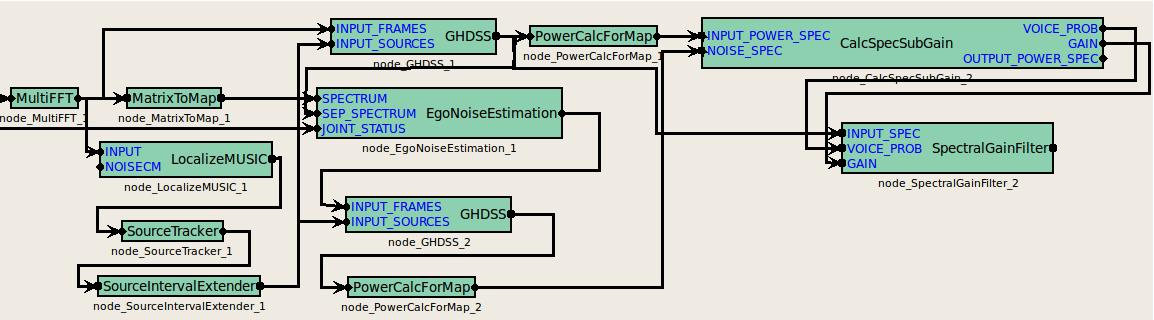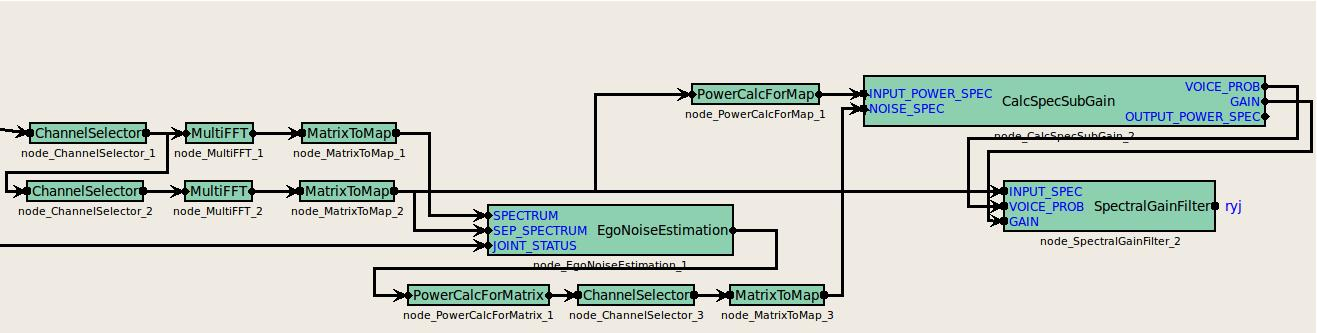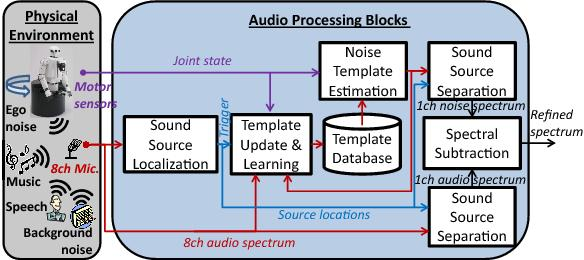# Overview¶

hark-ene includes the EgoNoiseEstimation module so as to estimate a robot’s ego-noise based on its status.

# Installation¶

1. Add HARK repository and install Basic HARK Packages. See HARK installation instructions for details.

2. Install HARK-Python

sudo apt-get install hark-ene

# EgoNoiseEstimation Node Description¶

## Outline of the node¶

EgoNoiseEstimation estimates ego noise by searching for a template most similar to the current ego noise spectrum from a database. Each template consists of a feature vector regarding the status of the motors of the robot, and a data vector containing the multi-channel spectrum of the ego noise. The templates are stored in a database. This node is able to read it from a file, re-create it, update it and write this database to a file. Node inputs are separated audio signals, complex spectrum of each channel, and joint status. The output is a multi-channel estimate of the noise spectrum.

## Necessary files¶

Corresponding parameter name Description
TEMPLATE_DB_FILENAME File containing template database.

## Usage¶

### When to use¶

This node is used when ego noise is required to be extracted. For this purpose, an input of joint status needs to be provided. The node computes the most similar template with a given joint status and sends the corresponding ego noise spectrum to the output. The inputs regarding the separated audio and the spectrum of each recorded channel are used for online learning of the templates.

### Typical connection¶The first figure shows a connection example for the EgoNoiseEstimation node. The node has three inputs as follows:

• SPECTRUM takes a multi-channel complex spectrum containing the recorded sounds as Map<int,ObjectRef> type,
• SEP_SPECTRUM takes the result of sound source separation,
• JOINT_STATUS takes the state of the motors from the robot.

There are two different ways to use this node. 1) Multi-channel ego noise suppression, and 2) Single-channel ego noise suppression.

The proposed usage of the multi-channel noise reduction system is illustrated in the first figure. The audio signals are firstly subject to Sound Source Localization (SSL) modules such as LocalizeMUSIC, and then to the ego noise estimation modules. In abstract implementation, the output of SSL is interpreted as a trigger in the template learning module to decide whether to apply a learning or a discarding process. (In physical implementation, however, the output of Sound Source Separation (SSS) modules such as GHDSS and Beamforming is connected to the input called SEP_SPECTRUM.) Also, the source locations constitute the input of SSS along with audio spectra to separate the useful audio signal, as well as of a second SSS module along with the estimated multi-channel noise template to separate the overall ego noise among all sound sources. By doing so, spectral subtraction can be applied on the audio spectrum of each individual sound source using its corresponding ego noise spectrum. The spectral subtraction is performed in a similar way as described for the HRLE module. As depicted in the first figure, the estimated noise output can be sent to CalcSpecSubGain and then to the SpectralGainFilter along with the outputs of GHDSS to suppress ego noise from the separated audio signals.If the node is used for single-channel ego noise suppression purpose, only a single channel of the estimates can be used as in the second figure. The input called SEP_SPECTRUM is a continuous single channel audio stream, which is basically the output of a ChannelSelector with a single channel. In a similar fashion the output of the EgoNoiseEstimation is fed to CalcSpecSubGain and SpectralGainFilter, thus single-channel ego noise suppression is achieved.

## Input-output and property of the node¶

Parameter list of EgoNoiseEstimation

Parameter name Type Default value Unit Description
FFT _LENGTH int 512 [pt] Analysis frame length.
NCHANNELS int 8   The number of input channels.
TEMPLATE _DB _FILENAME String     Filename of the existing template, no filename means no template database.
ENABLE _DEBUG bool false   Print debug messages.
SEARCH _WINDOW _WIDTH int 1   The number of best nearest neighbors, which are subject to a cross correlation (CC) in terms of their spectra. Most similar template in terms of its features and spectrum is selected. 1 means, CC is not applied.
NFEATURES int 64   Number of features used for nearest neighbor search (= # elements of joint status).
K int 10   Number of nearest neighbors (K-NN).
ENABLE _NORMALIZATION bool false   Normalize the joint-related information (features). The formula is $$F_{new}=(F_{old}-F_{min})/(F_{max}-F_{min}$$ ).
ENABLE _VEL _OVERWRITE bool false   Overwrite the velocities provided by the robot with the calculated values from positions.
FILTER _SIZE int 3   Filter size towards future (symmetric to the past). Works with ENABLE_VEL_OVERWRITE=true.
POSITION _WEIGHT float 1   Weight of the positions for NN distance calculation. Works with ENABLE_VEL_OVERWRITE=true.
VELOCITY _WEIGHT float 1   Weight of the velocities for NN distance calculation. Works with ENABLE_VEL_OVERWRITE=true.
ENABLE _LEARNING bool false   Update the internal template database using learning algorithms.
TEMPLATE _DB _NEW _FILENAME String     Filename of the new database to be stored. Works with ENABLE_LEARNING=true.
LEARNING _INTERVAL int 5   Time interval [in frames] to re-calculate the nearest neighborhood tree (must be greater than K). It is a trade-off between learning speed and computational power. Works with ENABLE_LEARNING=true.
TIME _CONSTANT float 10.0   Time constant (in frames) used in forgetting factor. Works with ENABLE_LEARNING=true.
ERROR _MARGIN float 0.0001   Error threshold for nearest neighbor search, which triggers the decision of a new template or update of an old template. Works with ENABLE_LEARNING=true.
ENABLE _NOISE _DETECTION bool false   True: Detects external directional noises and stops incremental learning, False: continuous incremental learning Works with ENABLE_LEARNING=true.
TILT _FEATURES float 10.0   Tilt value for the update formula in terms of feature similarity. Works with ENABLE_ADAPTIVE=true.
TILT _SPECTRA float 10.0   Tilt value for the update formula in terms of spectra similarity. Works with ENABLE_ADAPTIVE=true. hline

### Input¶

SPECTRUM
Map<int,ObjectRef> type. A pair containing the channel number and 1-channel complex spectrum of the recorded sound.
SEP_SPECTRUM
Map<int,ObjectRef> type. A pair containing the sound source ID of a separated sound and a 1-channel complex spectrum of the separated sound (Vector<complex<float> > type).
JOINT_STATUS
Matrix<float> type. It is a matrix (2N x 1) containing the angular positions (N) and velocities (N) of all joints.

### Output¶

ESTIMATED_NOISE
Matrix<complex<float> > type. Multi-channel complex spectra of the estimated ego noise. Rows correspond to channels, i.e., complex spectra of ego noise for each channel, and columns correspond to frequency bins.

### Parameter¶

FFT_LENGTH
int type. Analysis frame length, which must be equal to the values at a preceding stage value (e.g. AudioStreamFromMic or the MultiFFT node).
NCHANNELS
int type. Number of channels, which must be equal to the values at a preceding stage value (e.g. AudioStreamFromMic or the MultiFFT node).
TEMPLATE_DB_FILENAME
Filename of the existing template database to be loaded. The user has to give the root filename. The node tries to load one file with the filename “root+R” and one file with the filename of “root+I”, the files containing the real (R) and imaginary (I) templates respectively. These binary files are created in matlab. If there is no specified filename or the files cannot be found, the node returns just a warning message indicating it is starting to build the database from scratch. Please check the warning message carefully in the initialization phase. The file contains a header file with the size of the database (number of rows) and number of elements in each row. Each row of the matrix consists of features (joint status data) and the content (spectral data regarding each channel) of the template. Therefore the number of elements in each row is: nFeatures + nChannels * nFreqBins
ENABLE_DEBUG
bool type. Print debug messages about the estimation performance.
SEARCH_WINDOW_WIDTH
int type. The number of best nearest neighbors, which are subject to a cross correlation (CC) in terms of their spectra. Most similar template in terms of its features and spectrum is selected. 1 means, CC is not applied. SEARCH_WINDOW_WIDTH cannot be greater than K.
NFEATURES
int type. Number of features used in the templates. The size of the input JOINT_STATUS must be equal to the number of features labeling the templates.
K
int type. Number of nearest neighbors for K-NN search. The closest templates are selected based on the similarity of the joint status of templates.
ENABLE_NORMALIZATION
bool type. The default value is false. The user sets it to true, if the joint-related information (such as the positions and velocities) should be normalized so that they become [0 1]. The formula is $$F_{new}=(F_{old}-F_{min})/(F_{max}-F_{min})$$ . The $$F_{min}$$ and $$F_{max}$$ are fixed values, which indicate the position and velocity limits of each joint. These limits are embedded in the code.
ENABLE_VELOCITY_OVERWRITE
bool type. Overwrite the velocities provided by the robot with the calculated values from positions in this node. When true, select the FILTER_SIZE
FILTER_SIZE
int type. Filter size towards future (symmetric to the past). It is used to smooth the jerky velocity values.
POSITION_WEIGHT
int type. The user can determine the weight of the position features in the NN distance calculation.
VELOCITY_WEIGHT
int type. The user can determine the weight of the velocity features in the NN distance calculation.
ENABLE_LEARNING
bool type. This preference makes the learning methods active. The template database is updated using the data coming from the input SPECTRUM. If true, select the TEMPLATE_DB_NEW_FILENAME, LEARNING_INTERVAL, TIME_CONSTANT, ERROR_MARGIN, ENABLE_NOISE_DETECTION, and ENABLE_ADAPTIVE
TEMPLATE_DB_NEW_FILENAME
Filename of the new template database to be saved. The user just gives the root filename. The node tries to save one file with the filename of “root+R” and one file with the filename of “root+I”; the files containing the real and imaginary templates respectively. These binary files are intended to be read in Matlab. If there is no specified filename, the node does not write the internal template database to a file.
LEARNING_INTERVAL
int type. Time interval [in frames] to re-calculate and rebuild the nearest neighborhood tree (must be greater than K). During this time interval, the added or updated templates are stored in a temporary buffer. Only after the time has passed, the nearest neighborhood tree is rebuild. Thus, this parameter provides a trade-off between learning speed (quality) and computational power.
TIME_CONSTANT

float type. Time constant for smoothing (in frames). The formula is: forgettingFactor = exp( -dt/timeConstant). Given that dt = 1 frame, tc=0.2 $$\rightarrow$$ ff = 0.067; tc = 1 $$\rightarrow$$ ff= 0.36; tc=3 $$\rightarrow$$ ff = 0.75; by default: tc=10 $$\rightarrow$$ ff=

0.9; tc = 100 $$\rightarrow$$ 0.99. The higher the forgetting factor, the higher the contribution of the templates learned later
ERROR_MARGIN
int type. Error threshold for nearest neighbor, which triggers the decision of a new template or update of an old template. If the overall error is above the threshold, the current template is considered as a new template. Otherwise, it is treated as an existing template and is updated. Setting this value to 0 allows the continuous insertion of all incoming templates into the template database. Setting it to reasonable values above 0 enables the incremental learning. 0.0001 to 0.1 are reasonable values.
ENABLE_NOISE_DETECTION
bool type. If true, the second input called SEP_SPECTRUM is used to detect if there is a directional sound in the environment (The ego noise is a mostly diffuse noise source). If there is an existing directional source, the online learning system halts because the ego noise is contaminated with external sound sources, but the estimation system still continues to work. When the sound source stops emitting noise, the node starts learning the templates again.
bool type. Adaptive forgetting factor which makes the forgetting factor time variant. It overwrites the TIME_CONSTANT. True: Adaptive, False: Fixed. If true, select the TILT_FEATURES, TILT_SPECTRA
TILT_FEATURES
float type. Tilt value for the update formula in terms of feature similarity.
TILT_SPECTRA
float type. Tilt value for the update formula in terms of spectra similarity.

## Details of the node¶The outline of the basic architecture of the ego noise estimation system is shown in the flowchart in the third figure.

### Template Acquisition¶

The template generation method (the first block in the third figure) utilizes encoders of the $$J$$ number of joints, which measure the angular position ( $$\theta(l)$$ ) and velocity ( $$\dot{\theta}(l)$$ ) of each joint in every frame, $$l$$ . The resulting feature vector of the template has the form of $$\overrightarrow{F}(l)=[\theta_1(l),\dot{\theta}_1(l),\ldots,\theta_j(l),\dot{\theta}_j(l),\ldots,\theta_J(l),\dot{\theta}_J(l)]$$ . The feature vector is assigned to the ego noise spectral energy vector denoted as $$\overrightarrow{N}(l)$$ with $$N(k, l, m)$$ indicating the spectro-temporal energy in frequency bin $$k$$ , time frame $$l$$ , and the microphone (channel) $$m$$ . This data block, $$\overrightarrow{T}(l)=[\overrightarrow{F}(l):\overrightarrow{N}(l)]$$ , is called a parameterized template. In contrary to a setting as in the second figure, which was based on a single-channel data, having multiple channels ( $$m>1$$ ) allows us to separate ego noise with respect to the sound source it contaminates as in the first figure.

### Template Estimation¶

The core of this block is an instance-based, non-parametric classification technique known as the Nearest Neighbor (NN) algorithm. We used Approximate-Nearest Neighborhood (ANN) library . Basically, the spectral energy vector $$\overrightarrow{N}^x$$ stored in the template $$\overrightarrow{T}^x$$ of a large template database $$\boldsymbol{T}$$ with $$\overrightarrow{F}^x$$ having the shortest distance to $$\overrightarrow{F}(l)$$ and $$x$$ being the index of the $$x$$ -th element in the database is selected as the ego noise estimate $$\overrightarrow{\hat{N}}(l)$$ . How the template database $$\boldsymbol{T}$$ is created/updated is the function of the fourth block, template learning.

### Interfering Noise Detection¶

This block determines whether the current audio signal is corrupted by external noise sources, i.e., any sound except ego noise, such as music, speech, or the extracted template belongs to a pure ego noise signal. Keeping in mind that the ego noise behaves rather like a diffuse signal in the near-field, we put the interfering noise sources into two categories: directional and diffuse noise sources.

To detect the former type of sources, we use a multi-channel sound source localization method. In order to predict the Directions of Arrival (DoA) of sound sources, you may use any beamforming algorithm to determine the position and (more importantly to us) the existence of the directional sound source, if any.

If the noise is not a directional noise such as background noise, SSS is unable to detect it, but the explicit detection of the diffuse noise is not a crucial issue to the overall estimation system at all because the templates already contain the accumulated diffuse noise of background and ego noise. They can adapt to the changes in the diffuse noise by using the online template learning.

### Template Learning¶

The main goal of the last block of the third figure is to learn templates incrementally and include them to the template database. For this purpose, the output of the NN algorithm in the template estimation block is interpreted as a posteriori probability of the input pattern being the estimated pattern. Therefore, it provides a measure of similarity performance. This measure, also known as the relative confidence level, allows us to determine if each observed template is a previously known template or a new template to be learned. Based on the comparison of a given fixed distance threshold, $$d_t$$, with $$d_{min}(l)$$ having the smallest distance $$d(\overrightarrow{F}(l),\overrightarrow{F}^x)$$ in $$\boldsymbol{T}$$, the current template is either used to update the old template or it is inserted into the database as a new template. When the similarity is low, the template is treated as a missing template and inserted into $$\boldsymbol{T}$$; otherwise the adaptive update mechanism is active. In conjunction with the interfering noise detection block, this block builds up an autonomous and online learning system.

By setting ENABLE_ADAPTIVE to false, and the TIME_CONSTANT to 10.0, the contribution of past templates were reduced by introducing a fixed forgetting factor ( $$\eta=0.9$$ ), which computed the weighted average of the old and current template by laying the focus more on recently-acquired templates and less on earlier observations.

If you set ENABLE_ADAPTIVE to true, you introduce a time-variant forgetting factor to enhance the balance between adaptivity (learning quality) and stability (robustness against errors and unexpected transient noises, e.g., mechanical jittering and shuddering sounds). The former is achieved by using lower $$\eta(l)$$, whereas higher $$\eta(l)$$ enables stability. Its computation is as follows:

$\eta(l) =\frac{\frac{1}{1+\exp(-\sigma_1 d_{min}(l))}+\frac{1}{1+\exp(-\sigma_2 \epsilon(l))}}{2},$
$\epsilon(l) = \frac{\sum_{k=0}^K||N(k,l,1)|^2 - |\hat{N}(k,l,1)|^2|)}{\sum_{k=0}^K||N(k,l,1)|^2|},$

where $$\epsilon(l)$$ is the normalized noise estimation error of the first microphone signal (to reduce the computational cost, we selected $$m=1$$), $$\sigma_1$$ and $$\sigma_2$$ are tilt values for the sigmoid functions. So, $$\eta(l)$$ takes values between 0.5 and 1. When either the estimation error regarding the features, $$d_{min}(l)$$, or regarding the spectra, $$\epsilon(l)$$, is large, $$\eta(l)$$ increases. As a consequence, the contribution of the new erroneous template is reduced. The pseudo-code of the online learning algorithm is shown below.

Learning of templates

IF { $$d_{min}(\overrightarrow{F}(l),\overrightarrow{F}^x) \geq d_t$$ }

STATE $$[F^{new}(j,l):N^{new}(k,l,m)]\gets [F^{curr}(j,l):N^{curr}(k,l,m)]$$

ELSE

STATE $$[F^{old}(j,l):N^{upd}(k,l,m)]\gets [F^{curr}(j,l):\eta(l)N^{old}(k,l,m) + (1-\eta(l))N^{curr}(k,l,m)]$$

ENDIF